# Perl – DBI(Database Independent) Module | Set – 2

Perl allows the handling of Databases with the help of Perl Scripts. These scripts run with the help of a module known as DBI(Database Independent Interface) module. DBI module provides an API to interact with many databases such as MySQL, Oracle, etc. This module provides a set of variables and methods that provide interaction with a database interface and need not access the original database.

#### Creating a Table

To create a table in Perl, first we have to create a database in SQL. To do so, we will execute the following statement in SQL prompt:

`CREATE DATABASE EMP_DB;`

Now, a database by the name EMP_DB will web created. We will use this database to store record of employees in our database.

Now, we will connect to this database by using the following command –

`my \$dbh = DBI->connect("dbi:\$driver:\$dsn", \$username, \$passwd, { AutoCommit => 1 })`

Now, our connection to the database will be established and we will use the following statement to create a table, EMP_DEATAILS, to hold the record of employee’s details.

my \$sth = \$dbh->prepare(“CREATE TABLE EMP_DETAILS ( ID INT PRIMARY KEY, EMP_NAME VARCHAR(50), AGE INT, SALARY INT)”);
\$sth->execute();

The full program for reference is given below :

 `#!/usr/bin/perl -w ` `use` `strict; ` `use` `DBI; ` `my` `\$driver` `= ``"mysql"``; ` `my` `\$dsn` `= ``"database=EMP_DB"``; ` `my` `\$username` `= ``"root"``; ` `my` `\$passwd` `= ``""``; ` `my` `\$dbh` `= DBI->``connect``(``"dbi:\$driver:\$dsn"``,  ` `               ``\$username``, ``\$passwd``, ` `               ``{ ``AutoCommit` `=> 1 }) or  ` `               ``die` `"Failed to connect to database : \$DBI::errstr"``; ` `my` `\$sth` `= ``\$dbh``->prepare("CREATE TABLE EMP_DETAILS (  ` `                           ``ID INT PRIMARY KEY,  ` `                           ``EMP_NAME VARCHAR(50), ` `                           ``AGE INT, SALARY INT)"); ` ` `  `\$sth``->execute(); `

Output: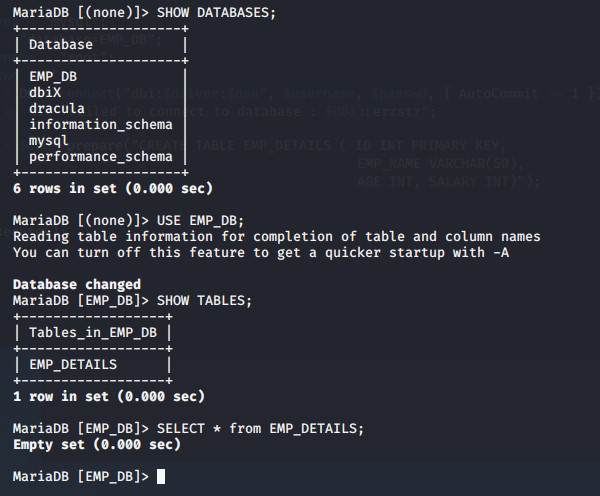#### Inserting in Database

Now that we have created EMP_DETAILS table in EMP_DB database, lets insert some values into the table by using the following statements :

 `#\$dbh->do("INSERT INTO EMP_DETAILS (ID, EMP_NAME, AGE, SALARY)   ` `VALUES (71, ``'CHINMAY'``, 23, 10000)")   ` `or ``die` `"Failed to insert :  \$DBI::errstr"``; ` ` `  `#\$dbh->do("INSERT INTO EMP_DETAILS (ID, EMP_NAME, AGE, SALARY)   ` `VALUES (73, ``'SANSKRUTI'``, 23, 15000)")   ` `or ``die` `"Failed to insert :  \$DBI::errstr"``; ` ` `  `\$dbh``->``do``("INSERT INTO EMP_DETAILS (ID, EMP_NAME, AGE, SALARY)   ` `VALUES (150, ``'DEREK'``, 37, 20000)")   ` `or ``die` `"Failed to insert :  \$DBI::errstr"``; ` ` `  `\$dbh``->``do``("INSERT INTO EMP_DETAILS (ID, EMP_NAME, AGE, SALARY)   ` `VALUES (200, ``'ROSS'``, 30, 24250)")   ` `or ``die` `"Failed to insert :  \$DBI::errstr"``; `

Output: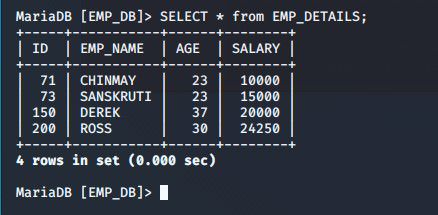#### Inserting Bind Values

Consider the below given program. In the program, we have used question marks(?) at some places. These question marks are known as placeholders. By using placeholders, the database driver can pre-process the query before executing it to the SQL prompt. This process is known as binding of data. Binding helps us in increasing the code security, which helps to prevent SQL injection attacks.

 `#!/usr/bin/perl -w ` `use` `strict; ` `use` `DBI; ` `my` `\$driver` `= ``"mysql"``; ` `my` `\$dsn` `= ``"database=EMP_DB"``; ` `my` `\$username` `= ``"root"``; ` `my` `\$passwd` `= ``""``; ` `my` `\$dbh` `= DBI->``connect``(``"dbi:\$driver:\$dsn"``,  ` `               ``\$username``, ``\$passwd``,  ` `               ``{ ``AutoCommit` `=> 1 }) or  ` `               ``die` `"Failed to connect to database : \$DBI::errstr"``; ` `  `  `# Inserting Bind Values ` `print` `"Enter Employee ID - "``; ` `my` `\$id` `= <>; ` `chomp``(``\$id``); ` `  `  `print` `"Enter Employee Name - "``; ` `my` `\$name` `= <>; ` `chomp``(``\$name``); ` `  `  `print` `"Enter Employee Age - "``; ` `my` `\$age` `= <>; ` `chomp``(``\$age``); ` `  `  `print` `"Enter Employee Salary - "``; ` `my` `\$salary` `= <>; ` `chomp``(``\$salary``); ` `  `  `my` `\$sth` `= ``\$dbh``->prepare("INSERT INTO EMP_DETAILS ( ` `                            ``ID, EMP_NAME, AGE, SALARY) ` `                            ``VALUES (?, ?, ?, ?)"); ` `                             `  `\$sth``->execute(``\$id``, ``\$name``, ``\$age``, ``\$salary``) or  ` `      ``die` `"Failed to insert : \$DBI::errstr"``; `

Output: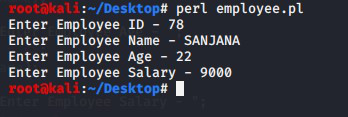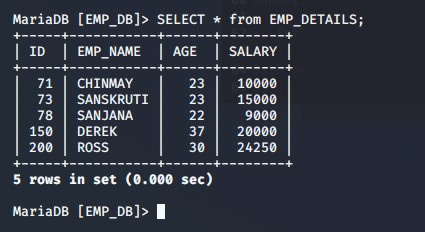Our database is now populated with some values. Now we need to read them back to the user. To do so we will use while loop to read back data to the user.

 `#!/usr/bin/perl -w ` `use` `strict; ` `use` `DBI; ` `my` `\$driver` `= ``"mysql"``; ` `my` `\$dsn` `= ``"database=EMP_DB"``; ` `my` `\$username` `= ``"root"``; ` `my` `\$passwd` `= ``""``; ` `my` `\$dbh` `= DBI->``connect``(``"dbi:\$driver:\$dsn"``,  ` `               ``\$username``, ``\$passwd``,  ` `               ``{ ``AutoCommit` `=> 1 }) or  ` `               ``die` `"Failed to connect to database : \$DBI::errstr"``; ` `  `  `my` `\$sth` `= ``\$dbh``->prepare(``"SELECT EMP_NAME, ID FROM EMP_DETAILS WHERE ID<100"``); ` `  `  `\$sth``->execute() or ``die` `"Failed to select rows : \$DBI::errstr"``; ` `  `  `my` `\$rows` `= ``\$sth``->rows; ` `print` `"Number of rows updated : \$rows \n"``; ` `  `  `while` `(``my` `@emp_data` `= ``\$sth``->fetchrow_array())  ` `{ ` `    ``my` `(``\$name``, ``\$id` `) = ``@emp_data``; ` `    ``print` `"Employee Name = \$name, Employee ID = \$id \n"``; ` `} ` `\$sth``->finish(); `

Output: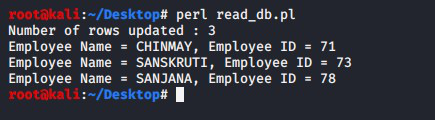#### Updating database values

To update any value of the table, we can use SQL UPDATE command in the following way :

 `#!/usr/bin/perl -w ` `use` `strict; ` `use` `DBI; ` `my` `\$driver` `= ``"mysql"``; ` `my` `\$dsn` `= ``"database=EMP_DB"``; ` `my` `\$username` `= ``"root"``; ` `my` `\$passwd` `= ``""``; ` `my` `\$dbh` `= DBI->``connect``(``"dbi:\$driver:\$dsn"``,  ` `               ``\$username``, ``\$passwd``,  ` `               ``{ ``AutoCommit` `=> 1 }) or  ` `               ``die` `"Failed to connect to database : \$DBI::errstr"``; ` `  `  `\$dbh``->``do``(``"UPDATE EMP_DETAILS SET ID=250 WHERE ID=150"``) ` `      ``or ``die` `"Failed to update : \$DBI::errstr"``; `

Output:

Original Table:Table after Data Updation: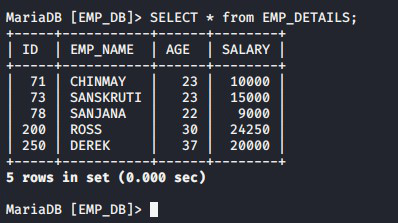#### Deleting database values

To delete a particular row from the table, we can use SQL DELETE statement in the following way :

 `#!/usr/bin/perl -w ` `use` `strict; ` `use` `DBI; ` `my` `\$driver` `= ``"mysql"``; ` `my` `\$dsn` `= ``"database=EMP_DB"``; ` `my` `\$username` `= ``"root"``; ` `my` `\$passwd` `= ``""``; ` `my` `\$dbh` `= DBI->``connect``(``"dbi:\$driver:\$dsn"``,  ` `            ``\$username``, ``\$passwd``, { ``AutoCommit` `=> 1 }) or  ` `            ``die` `"Failed to connect to database : \$DBI::errstr"``; ` ` `  `\$dbh``->``do``(``"DELETE FROM EMP_DETAILS WHERE ID=250"``) or  ` `     ``die` `"Failed to delete : \$DBI::errstr"``; ` `  `  `\$dbh``->``do``(``"DELETE FROM EMP_DETAILS WHERE EMP_NAME='ROSS'"``) or  ` `     ``die` `"Failed to delete : \$DBI::errstr"``; `

Output: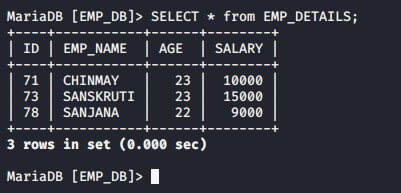Whether you're preparing for your first job interview or aiming to upskill in this ever-evolving tech landscape, GeeksforGeeks Courses are your key to success. We provide top-quality content at affordable prices, all geared towards accelerating your growth in a time-bound manner. Join the millions we've already empowered, and we're here to do the same for you. Don't miss out - check it out now!

Previous
Next